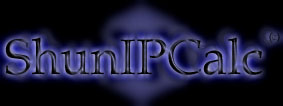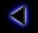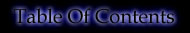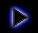.
How to convert decimal to binary ?
.
To convert decimal to binary, we first find the nearest power of 2 to the decimal number, then we substract it to the number. We do the same with the result of the substraction and so on until the result is 0.
.
For example, the decimal number 203 is in binary:

203-128=75 (128=2^7)
75-64=11 (64=2^6)
11-8=3 (8=2^3)
3-2=1 (2=2^1)
1-1=0 (1=2^0)

We put a "1" for each power of 2 "used during the calcul process" and "0" for each "not used" as follows:

 Value: 128 64 32 16 8 4 2 1 power of 2: 2^7 2^6 2^5 2^4 2^3 2^2 2^1 2^0 Binary number: 1 1 0 0 1 0 1 1
.
..
Copyright (c) 2002 - Guillaume Péan
www.shunsoft.net